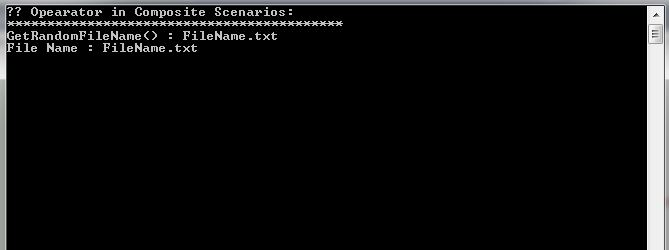?? Operator in C# is known as null coalescing operator, this is useful in scenario where you have to do a null check following with assignment. This operator provides a very easier way to set default value to a variable, it takes two operands, if the left operand is null, then it returns the right operand otherwise it returns left operand.

### ?? Operator syntax :

First Expression ?? Second ExpressionIf first expression is NULL , then evaluate the second expression. Otherwise evaluate first expression and ignore second expression)

For example:  i = j ?? k  // This implies, if j is null then assign k to i else assign j.

### ?? Opearator as assigning default value :

Following is an example of a null check and default value assignment.

In above, here left operand filePath value is null, that is why the assignment expression evaluates to the right operand and hence fileName is assigned to “DefaultFile.txt“.

Here left operand filePath value is not null, that is why the assignment expression evaluates to the left operand and the value “Invoice12345.text” assigned to variable fileName.

### Assigning default values example:

Lets look at some of the examples.

### ?? Opearator as short circuiting :

You can also use this operator in short circuiting scenarios as follows.

• If first expression is not null, then its value is the result of the assignment operation and the other expression is not evaluated.
• If first expression is null the value of second expression is the result of the assignment operation.

Lets look at below example, here FileName value will be based on their run time evaluated value.

### Short circuiting example :

When we run above example, it produces below output:In here, the short circuiting scenarios expression evaluates to “FileName.txt” . The first expression, the function  GetFileNameFromDb() returns null value and the next expression, the function GetDefaultFileName() returns value “FileName.txt”.

### ?? Operator in composite scenario :

You can also use this operator in composite scenarios . Lets look at below example.

Above chaining assignment expression evaluates to x, if x is not null else evaluates to y, if y is not null; otherwise it evaluates to z.

### Composite scenario example :

When we run above example, it produces below output:In here, the composite scenarios expression evaluates to “FileName.txt” .  The first expression, the function  GetFileNameFromDb() returns null value and the next expression, the function GetRandomFileName() returns value “FileName.txt”.

One thing to note here, In scenarios if method GetRandomFileName() also returns null value then the expression result will be “DefaultTextFile.txt”.